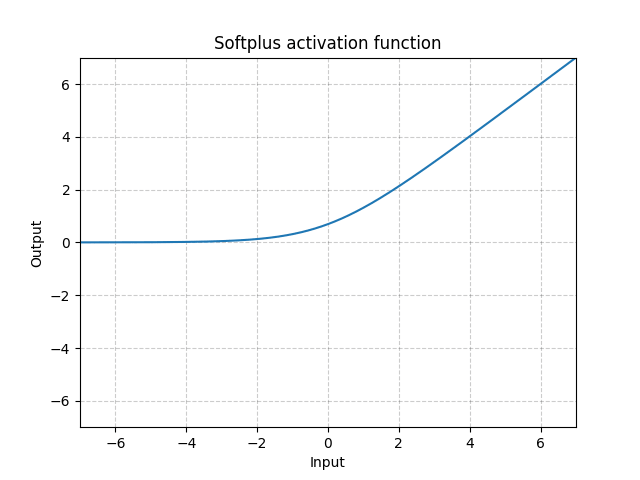/PyTorch

# Softplus

class torch.nn.Softplus(beta: int = 1, threshold: int = 20) [source]

Applies the element-wise function:

$\text{Softplus}(x) = \frac{1}{\beta} * \log(1 + \exp(\beta * x))$

SoftPlus is a smooth approximation to the ReLU function and can be used to constrain the output of a machine to always be positive.

For numerical stability the implementation reverts to the linear function when $input \times \beta > threshold$ .

Parameters
• beta – the $\beta$ value for the Softplus formulation. Default: 1
• threshold – values above this revert to a linear function. Default: 20
Shape:
• Input: $(N, *)$ where * means, any number of additional dimensions
• Output: $(N, *)$ , same shape as the inputExamples:

>>> m = nn.Softplus()
>>> input = torch.randn(2)
>>> output = m(input)


© 2019 Torch Contributors
Licensed under the 3-clause BSD License.
https://pytorch.org/docs/1.7.0/generated/torch.nn.Softplus.html